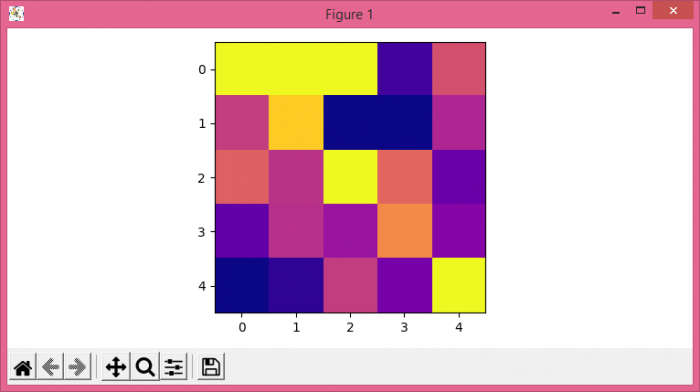# How to animate a time-ordered sequence of Matplotlib plots?

To animate a time-ordered sequence of Matplotlib plots, we can take the following steps −

• Set the figure size and adjust the padding between and around the subplots.
• Create a new figure or activate an existing figure.
• Add an axes to the figure as part of a subplot arrangement.
• Return the first recurrence after the given datetime instance using after() method.
• Write an animate() method to animate. Display the data as an image, i.e., on a 2D regular raster.
• To display the figure, use show() method.

## Example

import numpy as np
import matplotlib

matplotlib.use('TkAgg')
import matplotlib.pyplot as plt

plt.rcParams["figure.figsize"] = [7.50, 3.50]
plt.rcParams["figure.autolayout"] = True

fig = plt.figure()

def animate():
data = np.random.randn(5, 5)
im = plt.imshow(data, cmap="plasma")
for i in np.arange(1, 100):
data = np.random.randn(5, 5)
im.set_data(data)
fig.canvas.draw()
win = fig.canvas.manager.window
fig.canvas.manager.window.after(10, animate)
plt.show()

## Output